In :
import numpy as np
import matplotlib.pyplot as plt
%matplotlib inline
from IPython.display import Image
import pandas as pd


### Lecture 13:¶

• Learn a few more Pandas tricks
• Learn how to make more complicated plots with matplotlib
• Learn about the composition of the sun, solar system and Earth.
• Learn about exceptions in python

### More filtering tricks with Pandas¶

We now turn to some plots of elemental abundances in the Sun and the Solar System. I downloaded some data from Wikipedia (https://en.wikipedia.org/wiki/Abundances_of_the_elements_(data_page)) and processed them into a tab delimited file. Let's use the Unix command head to take a peek at the data.

In :
!head Datasets/ElementalAbundances/ElementalAbundances.txt

AtomicNumber	Symbol	Name	Solar	System
01	H	hydrogen	28000.0	28000.0
02	He	helium	2700.0	2700.0
03	Li	lithium	4e-07	5.7e-05
04	Be	beryllium	4e-07	7e-07
05	B	boron	1.1000000000000001e-05	2.1000000000000002e-05
06	C	carbon	10.0	10.0
07	N	nitrogen	3.1	3.1
08	O	oxygen	24.0	24.0
09	F	fluorine	0.001	0.0008500000000000001


The values are atomic mole fraction relative to Silicon so Si is 1.

The Solar System elemental abundances are from: Thomas J. Ahrens (ed.), Global Earth Physics : A Handbook of Physical Constants, American Geophysical Union (1995). ISBN 0-87590-851-9 Composition of the Solar System, Planets, Meteorites, and Major Terrestrial Reservoirs, Horton E. Newsom. Tables 1, 14, 15.

The values for the Sun are from: National Physical Laboratory, Kaye and Laby Tables of Physical & Chemical Constants (2005). Section 3.1.3, Abundances of the elements

We can read in this file with pd.read_csv( ) but this is not a 'comma separated variable' file (that is what the csv stands for) but a tab delimited text file. So, following the logic in Lecture 9 when we saved a file as a tab delimited format, we can set the separation method (sep) to tabs ('\t') for reading in data. And, we must tell Pandas that the header is in the first line (0).

Note that there are many ways of delimiting files, for example with spaces. That is why in Lecture 9 we used delim_whitespace=True (which we could also do here - try it yourself).

In :
Abundances=pd.read_csv('Datasets/ElementalAbundances/ElementalAbundances.txt',sep='\t',header=0)

Out:
AtomicNumber Symbol Name Solar System
0 1 H hydrogen 2.800000e+04 2.800000e+04
1 2 He helium 2.700000e+03 2.700000e+03
2 3 Li lithium 4.000000e-07 5.700000e-05
3 4 Be beryllium 4.000000e-07 7.000000e-07
4 5 B boron 1.100000e-05 2.100000e-05

### More Plotting techniques¶

We have successfully read in the file. Now let's plot 'AtomicNumber' versus the solar elemental abundances 'Abundances.Solar'.

In :
plt.plot(Abundances.AtomicNumber,(Abundances.Solar),'k-') # plot with a black line
plt.plot(Abundances.AtomicNumber,(Abundances.Solar),'ko') # put on some black dots
plt.xlabel('Atomic Number') # label the x axis
plt.ylabel('Abundance'); # label the y axis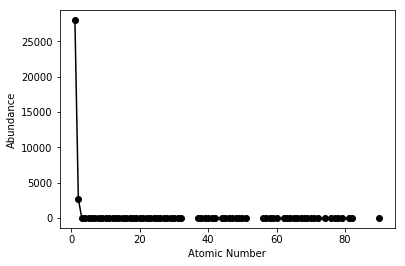Well that's odd - only the first two elements, hydrogen and helium, appear significant. The Sun is primarily H and He, so this is expected, but it isn't a very informative plot! We would like to see the other elements as well. We can do this by taking the logarithm of the abundances instead of the abundances. One way to do this is to use the NumPy function np.log10( ). Note that np.log( ) returns the natural log, while np.log10( ) returns the base 10 log.

In :
plt.plot(Abundances.AtomicNumber,np.log10(Abundances.Solar),'k-')
plt.plot(Abundances.AtomicNumber,np.log10(Abundances.Solar),'ko')
plt.xlabel('Atomic Number')
plt.ylabel('Log Abundance')
plt.title('Solar Elemental Abundances');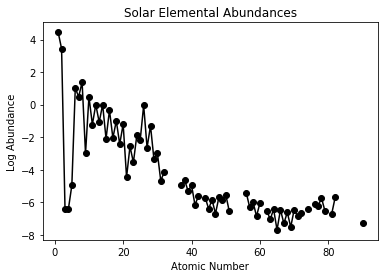That's better. While we are at it, there is another way to do this. We can set the y-scale to be a log, instead:

In :
plt.semilogy(Abundances.AtomicNumber,Abundances.Solar,'k-')
plt.semilogy(Abundances.AtomicNumber,Abundances.Solar,'ko')
plt.xlabel('Atomic Number')
plt.ylabel('Log Abundance')
plt.title('Solar Elemental Abundances');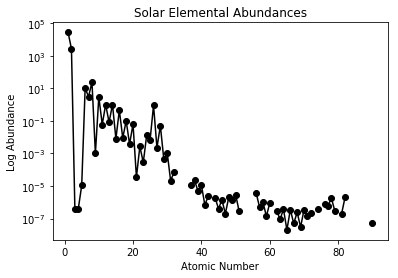Let's keep improving this plot. We could place the symbols above the points. To do this, we will convert the Series 'AtomicNumber' to a list, go through it with a for loop, and then plot each symbol above the dot by using our old frient plt.text( ) from Lecture 9.

To convert from a Pandas Series to a list, we use the method Series.tolist( ) as we learned in Lecture 9.

In :
print(Abundances.AtomicNumber.tolist()) #convert Series to list

[1, 2, 3, 4, 5, 6, 7, 8, 9, 10, 11, 12, 13, 14, 15, 16, 17, 18, 19, 20, 21, 22, 23, 24, 25, 26, 27, 28, 29, 30, 31, 32, 33, 34, 35, 36, 37, 38, 39, 40, 41, 42, 43, 44, 45, 46, 47, 48, 49, 50, 51, 52, 53, 54, 55, 56, 57, 58, 59, 60, 61, 62, 63, 64, 65, 66, 67, 68, 69, 70, 71, 72, 73, 74, 75, 76, 77, 78, 79, 80, 81, 82, 83, 84, 85, 86, 87, 88, 89, 90, 91, 92, 93, 94]


Now let's export the Symbols and abundances to lists as well.

In :
syms=Abundances.Symbol.tolist() # list of atomic symbols
print (syms)
Xs=Abundances.AtomicNumber.tolist() # list of atomic numbers
print (Xs)
Ys=Abundances.Solar.tolist() # list of solar abundances
print (Ys)

['H', 'He', 'Li', 'Be', 'B', 'C', 'N', 'O', 'F', 'Ne', 'Na', 'Mg', 'Al', 'Si', 'P', 'S', 'Cl', 'Ar', 'K', 'Ca', 'Sc', 'Ti', 'V', 'Cr', 'Mn', 'Fe', 'Co', 'Ni', 'Cu', 'Zn', 'Ga', 'Ge', 'As', 'Se', 'Br', 'Kr', 'Rb', 'Sr', 'Y', 'Zr', 'Nb', 'Mo', 'Tc', 'Ru', 'Rh', 'Pd', 'Ag', 'Cd', 'In', 'Sn', 'Sb', 'Te', 'I', 'Xe', 'Cs', 'Ba', 'La', 'Ce', 'Pr', 'Nd', 'Pm', 'Sm', 'Eu', 'Gd', 'Tb', 'Dy', 'Ho', 'Er', 'Tm', 'Yb', 'Lu', 'Hf', 'Ta', 'W', 'Re', 'Os', 'Ir', 'Pt', 'Au', 'Hg', 'Tl', 'Pb', 'Bi', 'Po', 'At', 'Rn', 'Fr', 'Ra', 'Ac', 'Th', 'Pa', 'U', 'Np', 'Pu']
[1, 2, 3, 4, 5, 6, 7, 8, 9, 10, 11, 12, 13, 14, 15, 16, 17, 18, 19, 20, 21, 22, 23, 24, 25, 26, 27, 28, 29, 30, 31, 32, 33, 34, 35, 36, 37, 38, 39, 40, 41, 42, 43, 44, 45, 46, 47, 48, 49, 50, 51, 52, 53, 54, 55, 56, 57, 58, 59, 60, 61, 62, 63, 64, 65, 66, 67, 68, 69, 70, 71, 72, 73, 74, 75, 76, 77, 78, 79, 80, 81, 82, 83, 84, 85, 86, 87, 88, 89, 90, 91, 92, 93, 94]
[28000.0, 2700.0, 4e-07, 4e-07, 1.1e-05, 10.0, 3.1, 24.0, 0.001, 3.0, 0.06, 1.0, 0.083, 1.0, 0.008, 0.45, 0.009000000000000001, 0.1, 0.0037, 0.064, 3.5000000000000004e-05, 0.0027, 0.00028, 0.013, 0.006900000000000001, 0.9, 0.0023, 0.05, 0.00045, 0.0011, 2.1e-05, 7.2e-05, nan, nan, nan, nan, 1.1e-05, 2.2000000000000006e-05, 4.9000000000000005e-06, 1.12e-05, 7e-07, 2.3e-06, nan, 1.9e-06, 4e-07, 1.4e-06, 2e-07, 2e-06, 1.3e-06, 3e-06, 3e-07, nan, nan, nan, nan, 3.8e-06, 5e-07, 1e-06, 1.3999999999999998e-07, 9.000000000000001e-07, nan, 3e-07, 9e-08, 3.7e-07, 2e-08, 3.5e-07, 5e-08, 2.4e-07, 3.0000000000000004e-08, 3.4e-07, 1.5e-07, 2.1e-07, nan, 3.6e-07, nan, 8e-07, 6e-07, 1.8000000000000001e-06, 3e-07, nan, 2e-07, 2e-06, nan, nan, nan, nan, nan, nan, nan, 5e-08, nan, nan, nan, nan]


As we saw from last lecture, we can use the Pandas method .dropna( ) to get rid of the 'nan's.

In :
Solar=Abundances.dropna(subset=['Solar']) # drop all the records with blank abundances
print (Solar.Solar.tolist())

[28000.0, 2700.0, 4e-07, 4e-07, 1.1e-05, 10.0, 3.1, 24.0, 0.001, 3.0, 0.06, 1.0, 0.083, 1.0, 0.008, 0.45, 0.009000000000000001, 0.1, 0.0037, 0.064, 3.5000000000000004e-05, 0.0027, 0.00028, 0.013, 0.006900000000000001, 0.9, 0.0023, 0.05, 0.00045, 0.0011, 2.1e-05, 7.2e-05, 1.1e-05, 2.2000000000000006e-05, 4.9000000000000005e-06, 1.12e-05, 7e-07, 2.3e-06, 1.9e-06, 4e-07, 1.4e-06, 2e-07, 2e-06, 1.3e-06, 3e-06, 3e-07, 3.8e-06, 5e-07, 1e-06, 1.3999999999999998e-07, 9.000000000000001e-07, 3e-07, 9e-08, 3.7e-07, 2e-08, 3.5e-07, 5e-08, 2.4e-07, 3.0000000000000004e-08, 3.4e-07, 1.5e-07, 2.1e-07, 3.6e-07, 8e-07, 6e-07, 1.8000000000000001e-06, 3e-07, 2e-07, 2e-06, 5e-08]


Now we have to re-generate our lists from the filtered DataFrame Solar

In :
syms=Solar.Symbol.tolist() # get the symbols exported to a list
Xs=Solar.AtomicNumber.tolist()
Ys=np.log10(Solar.Solar).tolist() # Don't forget to take the log value!


TIP: Another solution for getting rid of 'nan's would be replace them with zeros and then filter with the condition !=0 (remembering from Lecture 4 that 'not equal to' is '!=' in Python.

To replace blanks with a number, we can use the .fillna( ) method which does just that.

Now that we have rid ourselves of the blank values, we can label the points with the list of symbols using the plt.text( ) function.

We can tell plt.text( ) that we want the vertical alignment (va) of the data point to be on the bottom (va = 'bottom') and we want to center the horizontal alignment (ha). Here's how it goes.

In :
# do the usual
plt.plot(Solar.AtomicNumber,np.log10(Solar.Solar),'k-')
plt.plot(Solar.AtomicNumber,np.log10(Solar.Solar),'ko')
plt.xlabel('Atomic Number')
plt.ylabel('Log Abundance')
plt.title('Solar Elemental Abundances');
# now decorate the plot with symbol names
for k in range(len(syms)): # walk through the syms list
# here's how you do the notes:
plt.text(Xs[k],Ys[k],syms[k],va='bottom',ha='center',color='red')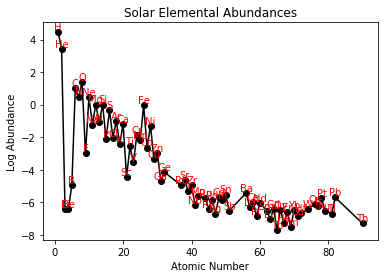Close - but the text labels are too close to the data points, so let's "scootch" them up. We can also rotate them as we did in Lecture 9. We can make the plot a bit bigger for clarity. Finally, we can set the fontsize using another keyword argument:

Also, it is now time learn about plt.figure(). This command creates a figure instance that has many useful methods, among them the ability to change the dimensions of the plot. This is done by passing a tuple with width and height as a parameter.

In :
plt.figure(figsize=(15,8))
plt.ylim(-8.5,6)
plt.plot(Solar.AtomicNumber,np.log10(Solar.Solar),'k-')
plt.plot(Solar.AtomicNumber,np.log10(Solar.Solar),'ko')
plt.xlabel('Atomic Number')
plt.ylabel('Log Abundance')
plt.title('Solar Elemental Abundances')
scootch=.5
for k in range(len(syms)):
plt.text(Xs[k],Ys[k]+scootch,syms[k],va='bottom',ha='center',color='red',rotation=90,fontsize=12)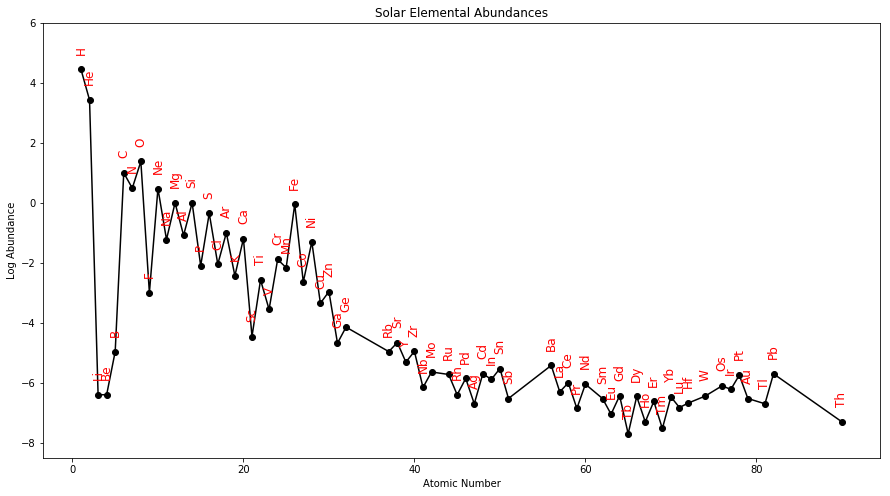Writing the labels above the points is one way to include the symbols. A different solution would be to write the labels on the X axis. We do that with plt.xticks( ). Setting the rotation keyword to 90 puts the tick labels on sideways (similar to how we did it for plt.text).

In :
plt.figure(figsize=(15,8))
plt.plot(Solar.AtomicNumber,np.log10(Solar.Solar),'k-')
plt.plot(Solar.AtomicNumber,np.log10(Solar.Solar),'ko')
plt.xlabel('Atomic Symbol')
plt.ylabel('Log Abundance')
plt.title('Solar Elemental Abundances')
plt.xticks(Xs,syms,rotation=90,fontsize=9,color='red');# see how we rotate the xticks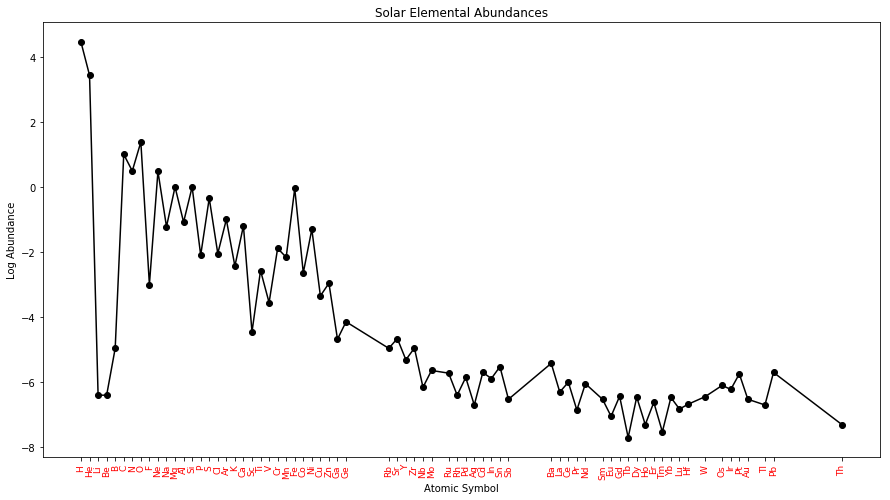Now let's look at the Solar System Abundances. We'll apply the same strategy.

In :
plt.figure(figsize=(15,8))
System=Abundances.dropna(subset=['System'])
syms=System.Symbol.tolist()
Xs=System.AtomicNumber.values.tolist()
Ys=np.log10(System.System).tolist() # Don't forget to take the log value!
plt.plot(Xs,Ys,'k-')
plt.plot(Xs,Ys,'ko')
plt.title('Solar System Elemental Abundances')
scootch=0.5
for k in range(len(syms)):
plt.text(Xs[k],Ys[k]+scootch,syms[k],va='bottom',ha='center',color='red',rotation=90,fontsize=12)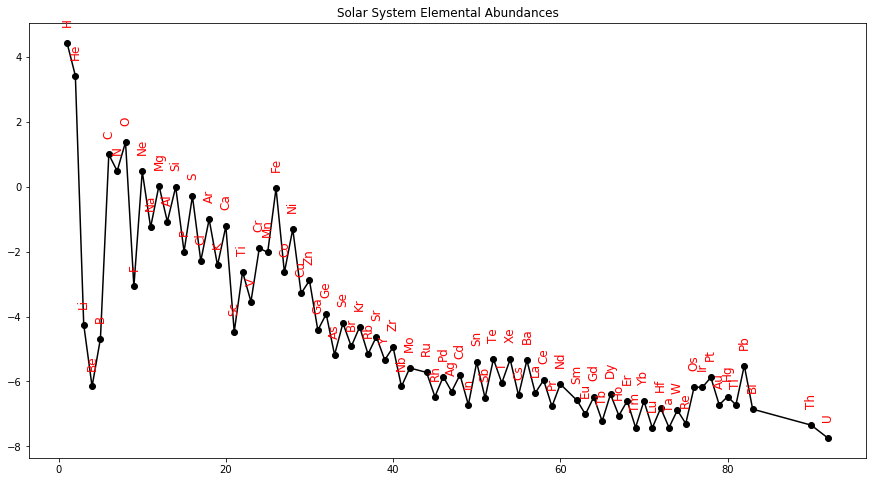Back to our elemental abundances, we'll take a look at one final example - the Earth's crust. You can find the data in: Datasets/ElementalAbundances/CrustalAbundances.txt

Let's take a peek:

In :
!head Datasets/ElementalAbundances/CrustalAbundances.txt

AtomicNumber	Symbol	Name	Crust	SiNormalized
1	H	hydrogen	0.0014	0.004964539
2	He	helium	8.00E-09	2.84E-08
3	Li	lithium	2.00E-05	7.09E-05
4	Be	beryllium	2.80E-06	9.93E-06
5	B	boron	1.00E-05	3.55E-05
6	C	carbon	0.0002	0.00070922
7	N	nitrogen	1.90E-05	6.74E-05
8	O	oxygen	0.461	1.634751773
9	F	fluorine	0.000585	0.002074468


And read, filter and plot as before.

In :
plt.figure(figsize=(15,8))
Crust=CrustalAbundances.dropna(subset=['SiNormalized']) # drop the blanks
syms=Crust.Symbol.tolist() # export the Symbol Series to syms
Xs=Crust.AtomicNumber.tolist()
Ys=np.log10(Crust.SiNormalized).tolist() # Don't forget to take the log value!
plt.plot(Xs,Ys,'k-')
plt.plot(Xs,Ys,'ko')
plt.title('Crustal Elemental Abundances')
scootch=.5 # pad the Y axis with scootch
for k in range(len(syms)):
plt.text(Xs[k],Ys[k]+scootch,syms[k],va='bottom',ha='center',color='red',rotation=90,fontsize=12)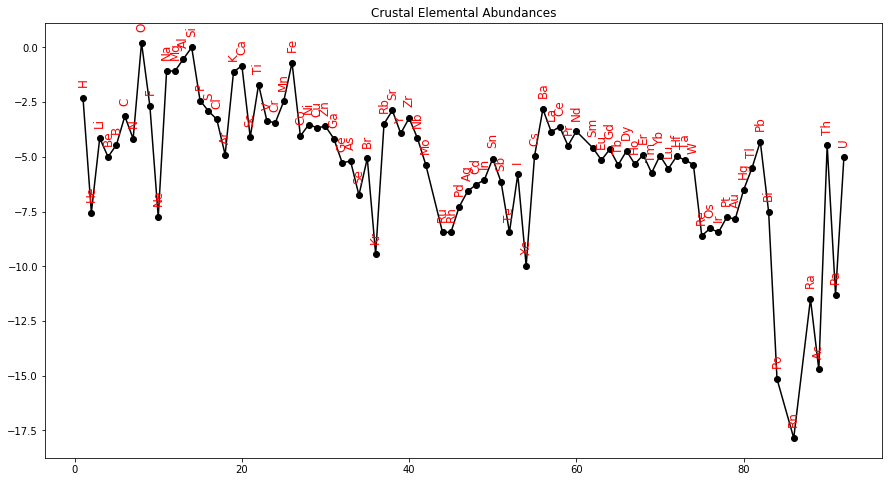Wow! that looks different! Where did all the Hydrogen and Helium go?

To get a better comparison among the resevoirs, it would be handy to plot all three images on one figure. There are many ways to accomplish this, for example making a stacked plot with the figures on top of each other, or three separate figures side-by-side. Here we want three figures stacked vertically.

Up to now we have just used plt.figure( ) to adjust the size of the default plot. But there is a lot more to a plt.figure( ) instance that we can use. To benefit from that, we make a figure instance like so:

In :
fig=plt.figure(1,(5,15)) # figure instance

<Figure size 360x1080 with 0 Axes>

The fig instance has many methods. One of these is fig.add_subplot(row,column,plot_number).

In this case, we want 3 rows in one column and we will add subplots in order (1-3).

In :
fig=plt.figure(1,(15,15)) # figure instance
fig.add_subplot(3,1,1) # the 3,1,1 means three rows, one column,  and this is the first plot.
plt.plot(Solar.AtomicNumber,np.log10(Solar.Solar),'k-')
plt.plot(Solar.AtomicNumber,np.log10(Solar.Solar),'ko')
plt.title("Solar Elemental Abundances")
plt.ylabel('Log Abundance')
fig.add_subplot(3,1,2) # this makes the second plot of three in one column
plt.plot(System.AtomicNumber,np.log10(System.System),'k-')
plt.plot(System.AtomicNumber,np.log10(System.System),'ko')
plt.title("Solar System Elemental Abundances")
plt.ylabel('Log Abundance')
fig.add_subplot(3,1,3) # and here comes the third plot.
plt.plot(Crust.AtomicNumber,np.log10(Crust.Crust),'k-')
plt.plot(Crust.AtomicNumber,np.log10(Crust.Crust),'ko')
plt.title("Crustal Elemental Abundances")
plt.ylabel('Log Abundance')
plt.xlabel('Atomic Number'); # the xlabel comes here because the 3rd plot is the lowest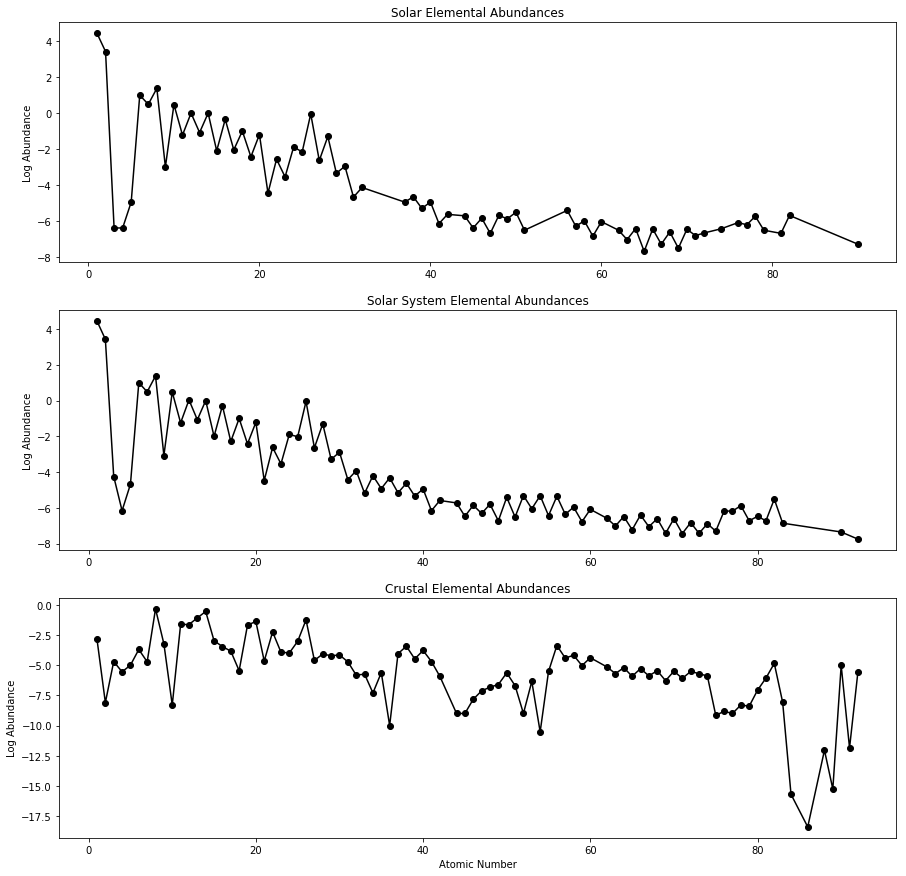Other ways of setting up plots are possible and we will learn about some of them in later lectures. If you are interested, see: http://matplotlib.org/api/pyplot_api.html.

How else can we represent the crustal abundance data? Some folks love pie charts. We can create a pie chart of the most abundant elements in the crust. To do this, we should probably filter for the most abundant elements.

So we'll begin by sorting the Crustal abundances into descending order. Remember from last lecture that we can use sort_values( ) to do this.

In :
Crust.sort_values(by="Crust", ascending=False).head(14) # the head method gives us the top 14.

Out:
AtomicNumber Symbol Name Crust SiNormalized
7 8 O oxygen 0.461000 1.634752
13 14 Si silicon 0.282000 1.000000
12 13 Al aluminium 0.082300 0.291844
25 26 Fe iron 0.056300 0.199645
19 20 Ca calcium 0.041500 0.147163
10 11 Na sodium 0.023600 0.083688
11 12 Mg magnesium 0.023300 0.082624
18 19 K potassium 0.020900 0.074113
21 22 Ti titanium 0.005650 0.020035
0 1 H hydrogen 0.001400 0.004965
14 15 P phosphorus 0.001050 0.003723
24 25 Mn manganese 0.000950 0.003369
8 9 F fluorine 0.000585 0.002074
55 56 Ba barium 0.000425 0.001507

Here we see that abundances less than about 1% are trivial, so let's filter 'Crust' for values > 0.01.

In :
Top=Crust[Crust.Crust>.01] # this takes all the values with Crust >0.01
Top

Out:
AtomicNumber Symbol Name Crust SiNormalized
7 8 O oxygen 0.4610 1.634752
10 11 Na sodium 0.0236 0.083688
11 12 Mg magnesium 0.0233 0.082624
12 13 Al aluminium 0.0823 0.291844
13 14 Si silicon 0.2820 1.000000
18 19 K potassium 0.0209 0.074113
19 20 Ca calcium 0.0415 0.147163
25 26 Fe iron 0.0563 0.199645

And now, drum roll please, for the pie chart. We can use plt.pie( ) to make the chart. It has a keyword argument labels= which takes a list. This allows us to label all the slices with a list of the symbols as shown here:

In :
plt.pie(Top.Crust,labels=Top.Symbol.tolist()) # see the labels trick?
plt.axis('equal'); # for some reason the pie is not round by default.  this makes it round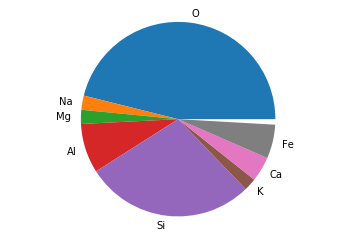But there is a slice out of our pie! This is because after removing the 'trivial' elements, they no longer t sum to 1. Let's renormalize them. To do that, we must first sum up all the values, then divide them all by the sum.

In :
total= Top.Crust.sum() # the sum() method takes the sum of the Series - duh.
Normalized=Top.Crust/total # makes a Series that is normalized by the sum
syms=Top.Symbol.tolist()
plt.pie(Normalized,labels=syms)
plt.axis('equal');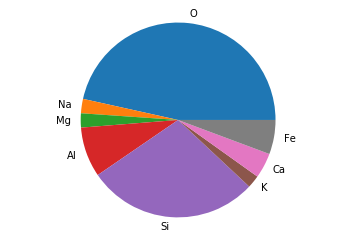Some people love pie charts while others like bar charts better. So let's look at the data as a bar chart.

In :
nums=Top.AtomicNumber.tolist() # get a list of Atomic Numbers
plt.bar(nums,Normalized) # this makes the bar chart
plt.xticks(visible=False) # HA!  we can turn the x tick labels off!

for k in range(len(syms)): # and label the bars this way instead
plt.text(nums[k] ,Normalized.tolist()[k],syms[k],va='bottom',ha='center')
# notice how we had to turn the Series Normalized into a list for this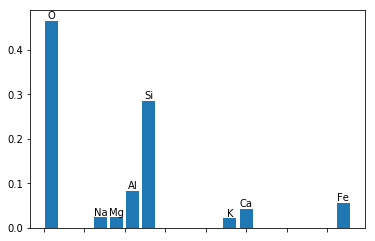### Exceptions¶

It's time for a little chat about bugs. We all have them. Nothing to be ashamed of. But you need to know how to deal with them. How should your code handle errors? Should it notify the user? Stop working entirely? Ignore the error and continue? You can control how your code responds to errors, by catching exceptions (errors) and handling them however you choose.

Have you noticed that whenever you write improper code, the error type is defined? For example, the cell below throws a NameError because we haven't defined the variable abcdef.

In :
print (abcdef)
print ("complete")

---------------------------------------------------------------------------
NameError                                 Traceback (most recent call last)
<ipython-input-28-ceca4aff99ce> in <module>
----> 1 print (abcdef)
2 print ("complete")

NameError: name 'abcdef' is not defined

The error crashes the code and prevents any of the following commands from executing. We could catch this exception and allow the program to continue. The syntax for exceptions is as follows:

try:

statement



except ( ExceptionType):

statement



The first statement is attempted. If that statement throws an exception and the exception is included in ExceptionType, then the second statement is executed and the program continues.

Here's our original example, but this time, we'll catch any NameError exceptions:

In :
try:
print (abcdef)
except(NameError):
print ('This variable does not exist')

print ("See I didn't crash!  I just told you what went wrong!")

This variable does not exist
See I didn't crash!  I just told you what went wrong!


Here's another example:

In :
try:
print (abcdef)
except Exception as inst: # call the Exception instance inst
#print (Exception.__class__.__name__)
print (type(inst)) # print the type of Exception
print (inst.args) # print any arguments of the instance
print (inst) # print out the error message

<class 'NameError'>
("name 'abcdef' is not defined",)
name 'abcdef' is not defined


These messages give you a clue how to fix the problem (in this case, by defining abcdef). These clues can be used for various debugging tricks like what to do for different types of errors.

In :
try:
print (abcdef)
except KeyError:
print ('I could do one thing here')
except NameError:
print ('I could do something else here')

I could do something else here